# [境外游] 俄罗斯旷野之旅----野外狩猎

 【俄罗斯狩猎】野性俄罗斯狩猎9日之旅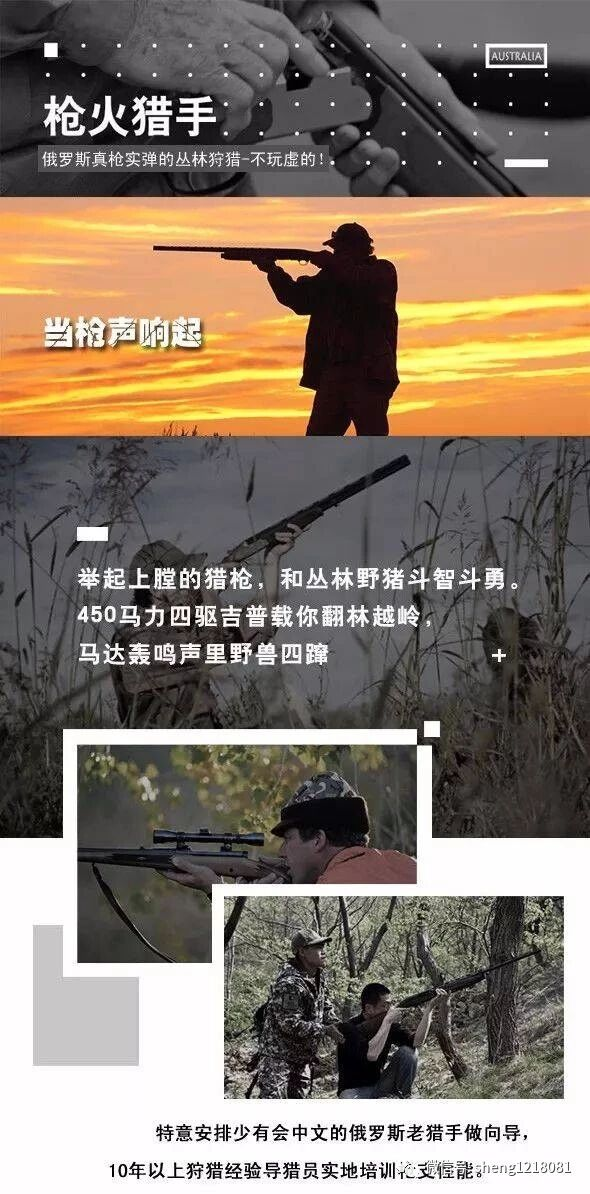[size=0.48] 第一天 徐州--哈尔滨（火车票自理）[size=0.48]第二天哈尔滨-海拉尔（火车票自理。）也可直飞海拉尔当地集合（机票自理）[size=0.48]大交通自理，到达海拉尔——“草原明珠”。[size=0.48]飞机到的免费接机，安排入住（含），晚餐呼伦贝尔火锅涮羊肉（含）[size=0.48]交通:汽车（含）[size=0.48]餐：含晚餐[size=0.48]住宿：准四星宾馆[size=0.48]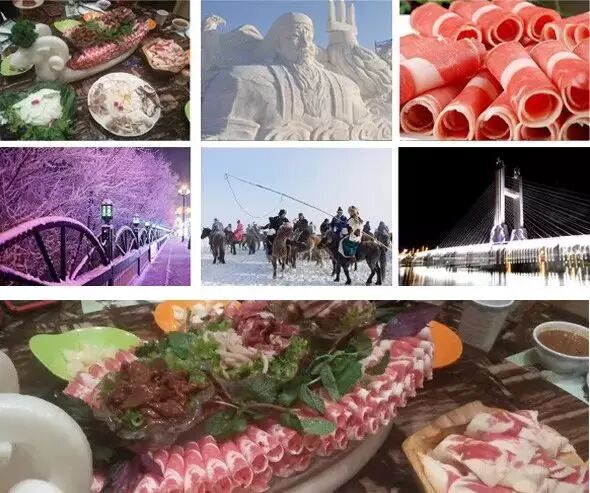[size=0.48]第三天 上午统一安排车辆去海拉尔东山国家机场飞赤塔（含机票）[size=0.48]（起飞航班：上午11点-12点）（俄罗斯赤塔落地航班：下午1点-2点到达赤塔）[size=0.48]安排赤塔宾馆入住，赤塔游览：枪店、列宁广场、圣母教堂等名胜古迹[size=0.48]交通:飞机（含）[size=0.48]餐：含早餐[size=0.48]住宿：赤塔宾馆[size=0.48]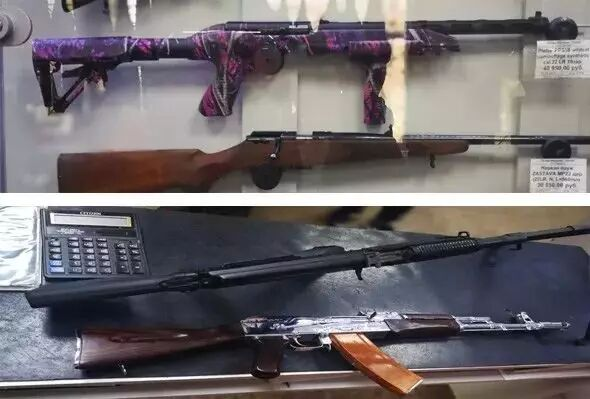[size=0.48]第四天 出发猎场—猎场武器使用及射击训练--参观西伯利亚马鹿基地[size=0.48]早餐后出发猎场，我们经验丰富的向导-托利亚会指导大家如何安全正确地使用武[size=0.48]器，在狩猎中安全使用武器的方法，并且指导在哪些地方可以向站立或者奔跑的野生[size=0.48]动物射击，如何上子弹。直观地向大家展示立正与支撑时射击的要领。学习指导过[size=0.48]后，我们每一位客户都有机会带着武器在实践中演练，此后方能共同出发狩猎[size=0.48]猎场晚餐（含），俄罗斯桑拿浴（含）[size=0.48]交通:俄罗斯汽车[size=0.48]餐：早餐、午餐、晚餐[size=0.48]住宿：猎场木屋4-6人一屋[size=0.48]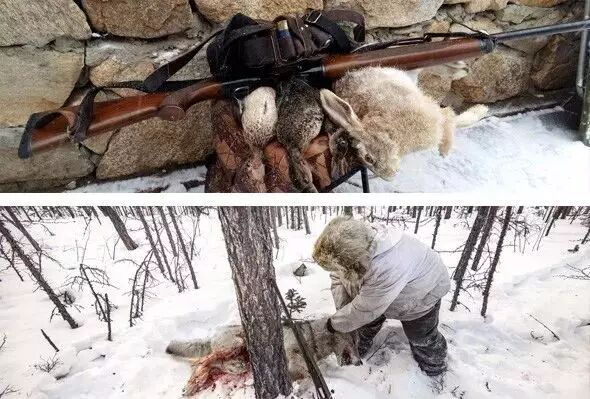[size=0.48]第五天 原始森林狩猎[size=0.48]早餐后乘坐装甲车和摩托车出发狩猎，一整天都安排狩猎，[size=0.48]经验丰富的猎人将组织麋鹿和野猪的围猎，根据实际情况，午餐可能在野外进行或者[size=0.48]回到基地后用餐，围猎活动会根据某个狩猎场动物多少分成几个阶段。回到基地后会[size=0.48]给我们准备用刚刚打回来的野味做的菜，如果时间允许，您还可以骑上越野摩托车环[size=0.48]绕狩猎场的四周观光。[size=0.48]晚上是晚餐和洗桑拿[size=0.48]交通：汽车: 装甲车，摩托车，UAZ-452[size=0.48]餐：含早餐、中餐、晚餐[size=0.48]住宿：猎场木屋 4-6 人一屋[size=0.48]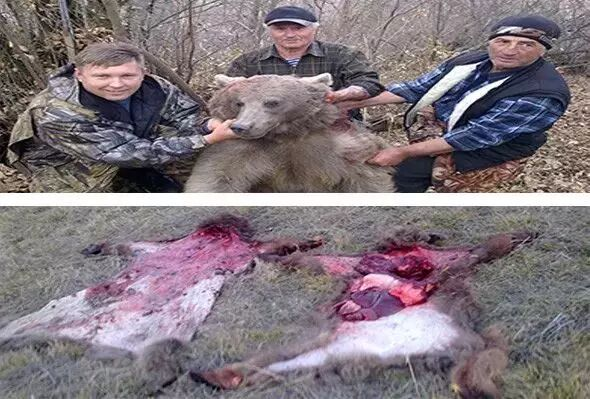[size=0.48]第六天 松林狩猎—尝试不同武器的射击方法—猎人餐厅丰盛的晚宴[size=0.48]一大早我们就出发去松林狩猎，围猎，实地查看野猪和麋鹿栖息地。大概下午四点左[size=0.48]右返回基地，剩下的时间我们尝试不同武器的射击方法，骑越野摩托车并租用越野[size=0.48]车。晚餐是猎人餐厅丰盛的晚宴，返回市里，宾馆住宿[size=0.48]交通汽车: 装甲车，摩托车，UAZ-452[size=0.48]餐：含早餐、中餐、晚餐[size=0.48]住宿：赤塔[size=0.48]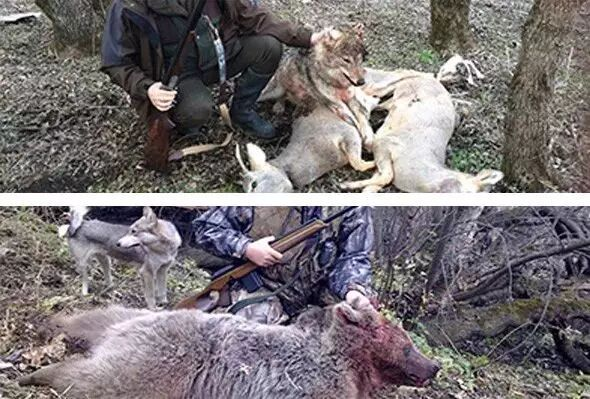[size=0.48]第七天赤塔机场——海拉尔东山国际机场，晚火车到哈尔滨[size=0.48] [size=0.48]上午自由活动，下午1点赤塔国际机场飞回海拉尔东山国际机场，解散，当天飞[size=0.48]机不能回的食宿自理[size=0.48]交通:飞机（含）[size=0.48]餐：含早餐[size=0.48]第八天哈尔滨--徐州[size=0.48]第九天到达徐州[size=0.48]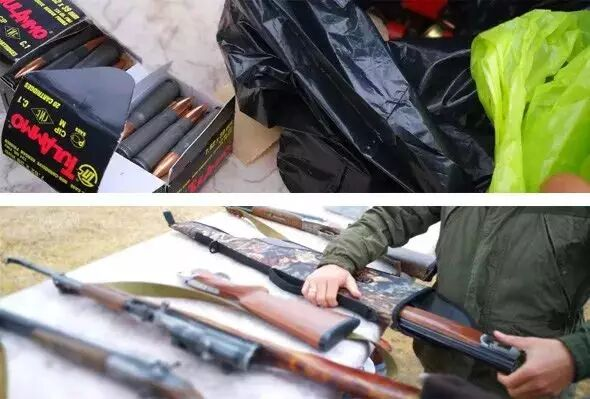[size=0.48]行程排期[size=0.48]12月25——1月2[size=0.48][size=0.48]出行须知[size=0.48]1、鞋：专业的登山鞋或雪地鞋最好。写的防滑性一定要尽量好，因为路上会有[size=0.48]冰坡，很滑很难走；[size=0.48]2、袜子：越厚越好； [size=0.48]3、帽子：一定要能护住耳朵的； [size=0.48]4、手套：准备薄款抓绒手套、一双厚棉手套（因为带着厚手套拍照不方便，就 [size=0.48]用薄手套）；[size=0.48]5、围巾、口罩、头巾带着备用； [size=0.48]6、上衣：保暖内衣+羊绒衫+抓绒衣+羽绒服等，是个人体制和当天气温情况能 [size=0.48]减则减（泡温泉自带泳衣泳裤）；[size=0.48]7、裤子：防水、不粘雪的面料为佳（保暖裤、羽绒裤、冲锋裤等）； [size=0.48]8、雪套：有就带着； [size=0.48]9、墨镜：必带（防雪盲）； [size=0.48]10、头灯或手电：必带； [size=0.48]11、包：建议一个拉杆箱，一个小背包（随身携带、脱换衣物、方便穿越） [size=0.48]12、相机/手机：充电设备带齐、充电宝必带； [size=0.48]13、自己带一些可以增加热量的东西，如巧克力架、朱古力等，保温水壶，方 [size=0.48]便在路上喝热水；[size=0.48]14、准备些热贴，手机相机冷的时候能用。 [size=0.48]15、俄罗斯冬天很冷，多穿，合理搭配。 [size=0.48][size=0.48]报名须知[size=0.48]1、提前一周提供本人护照及**原件的复印件或彩色扫描件，并交齐全款。[size=0.48]2、狩猎活动因俄方需提前确定人数及预留狩猎场，我方需提前上交费用，所以[size=0.48]报名完成后如因个人原因不能参加此次行程的队员费用一律不退。[size=0.48]3、要求身体健康，没有重大疾病以及慢性病[size=0.48]4、无论任何线路为所有成员强制购买户外保险（购买过户外年险并在保期内的[size=0.48]人除外），请配合将相关信息提供给对应的工作人员。[size=0.48]5、报名以达到人数上限或到达截止时间自动关闭报名！[size=0.48]6、请伙伴们看好时间，地点，准时出发，迟到者视主动放弃，不接受任何理[size=0.48]由，不退还任何费用。 [size=0.48]费用包含[size=0.48]1、签证费用[size=0.48]2、保险费用[size=0.48]3、全国各地往返海拉尔接送机/站[size=0.48]4、海拉尔东山国际机场往返俄罗斯赤塔机场机票费用[size=0.48]5、狩猎费用（猎人、**、子弹、装备、 装甲车，摩托车，UAZ-452）[size=0.48]6、全程向导、领队、翻译费用[size=0.48]7、俄罗斯赤塔当地车费[size=0.48]8、行程内包含的餐费7正5早（全程向导、领队、翻译随队就餐）[size=0.48]9、全程住宿费用[size=0.48]1.第一晚：海拉尔准四宾馆（标间）[size=0.48]2.第二晚：俄罗斯赤塔宾馆（标间）[size=0.48]3.第三晚：狩猎场（4-6人猎人木屋）[size=0.48]4.第四晚：狩猎场（4-6人猎人木屋）[size=0.48]5.第五晚：俄罗斯赤塔宾馆（标间）[size=0.48]参加此次行程的国内往返大交通[size=0.48]餐费不包含第二日赤塔午餐、晚餐，第六日午餐、晚餐[size=0.48]注意事项[size=0.48]一、出关[size=0.48]1. 护照和签证是公民出境在国外的唯一有效**明。丢失护照是最麻的事[size=0.48]。在出入国境时，护照由本人自行保管比较方便，其它时间可由团队统一保[size=0.48]存，每人身上可存护照、签证复印件，以备查。万一护照丢失，除报警外，要[size=0.48]到我国在当地使、领馆**为此，请准备三张2寸照片），方能回[size=0.48]国。[size=0.48]2、请提前确认机票的日期和航班，登机前问清登机时间与门号。按国际惯例[size=0.48]，搭乘国际航班团队，要提前2.5-3小时到机场办理登机及托运手续。搬运行李[size=0.48]以每人一件（限二十公斤）最佳。[size=0.48]请注意：按我国外汇管理局规定，出国人员可携带最多不超过5000美元的等值[size=0.48]货币出境[size=0.48]二.、乘飞机注意事项[size=0.48]1、行李分为托运行李与随身行李。托运行李不得超过23公斤，随身行李大小不[size=0.48]得超过22寸x14寸x4寸。若带有小剪刀、指甲剪之类的物品，只能托运，严禁随[size=0.48]身携带；[size=0.48]2、注意保管好旅行证件及个人财物。托运行李中不得夹带现金。[size=0.48]3、务请遵守各国法律法规，严禁携带牟利私货及违例物品，否则后果自负；[size=0.48]4、机场内只有在吸烟室或吸烟区才可以吸烟；[size=0.48]5、在机场出关时一定要注意行李安全，看好托运行李，同时拎好手提行李，各[size=0.48]人的重要物品（如护照、签证、机票、现金等）要由自己妥善保管。[size=0.48]三、欧洲入境须知[size=0.48]1.旅行自备：[size=0.48]请随身携带自用洗漱用具（包括：洗发液、沐浴露、牙具、毛巾等）、拖鞋、[size=0.48]雨具、相机、电池、太阳镜、护肤品。请根据自身身体状况要携带所需物品。[size=0.48]2、信用卡：[size=0.48]American Express Card, Diners Club, VISA, Master Card 等等信用卡；在欧洲[size=0.48]的酒店，商店，银行，及餐厅均可使用信用卡付帐，也可用于购买机票及租[size=0.48]车，但一般不能用于火车、公共汽车及轮渡上。[size=0.48]3、通讯[size=0.48]从欧洲国家拨打中国的电话，都是先拨0086，再加上地区号和电话号码即可[size=0.48]。在欧洲的酒店房间内拨打电话通常较为昂贵，建议购买欧洲通用的电话卡[size=0.48]，那样将经济得多。中国电信、联通、移动网络的手机需提前开启国际漫游功[size=0.48]能才可使用。[size=0.48]4、吸烟：[size=0.48]欧洲国家在公共场所执行严格的禁烟令。除了酒吧以及餐馆的吸烟区，在所有[size=0.48]的室内场所吸烟都是违反国家法律的，违反者将被处以重罚。[size=0.48] [size=0.48]【自由活动活动安全告知】[size=0.48]1、**使用必须遵从职业猎人指导，不可随意用枪，不可藏匿枪械及子弹，如[size=0.48]因擅自用枪及藏匿[size=0.48]2、枪械及子弹导致的一切后果由个人承担，并追究其法律责任。[size=0.48]3、自由活动、参加自费活动时，请注意人身安全和财物安全，需提前告知领[size=0.48]队，不可擅自出行。[size=0.48]如因擅自出行所导致的一切后果由个人承担，并追究其法律责任。[size=0.48]4、自由活动期间，如需购物时，请注意商品质量及价格，应细心鉴别商品真[size=0.48]伪。[size=0.48]5、自由活动期间须遵守当地的法律法规及风俗习惯咨询电话：18905207293，微信13813292991
 本版积分规则 回帖后跳转到最后一页